# NCERT Solution - Structure of Atom, Class 9 Science Notes - Class 9

## Class 9: NCERT Solution - Structure of Atom, Class 9 Science Notes - Class 9

The document NCERT Solution - Structure of Atom, Class 9 Science Notes - Class 9 is a part of Class 9 category.
All you need of Class 9 at this link: Class 9

Page No: 47

1. What are canal rays?

Canal rays are positively charged radiations that can pass through perforated cathode plate. These rays consist of positively charged particles known as protons.

2. If an atom contains one electron and one proton, will it carry any charge or not?

An electron is a negatively charged particle, whereas a proton is a positively charged particle. The magnitude of their charges is equal. Therefore, an atom containing one electron and one proton will not carry any charge. Thus, it will be a neutral atom.

Page No: 49

1. On the basis of Thomson's model of an atom, explain how the atom is neutral as a whole.

As per Thomson’s model of the atom, an atom consists both negative and positive charges which are equal in number and magnitude. So, they balance each other as a result of which atom as a whole is electrically neutral.

2. On the basis of Rutherford's model of an atom, which subatomic particle is present in the nucleus of an atom?

On the basis of Rutherford's model of an atom, protons are present in the nucleus of an atom.

3. Draw a sketch of Bohr's model of an atom with three shells.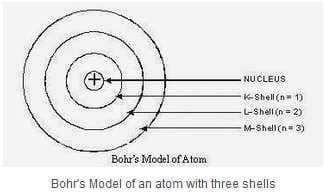4. What do you think would be the observation if the α-particle scattering experiment is carried out using a foil of a metal other than gold?

If α-particle scattering experiment is carried out using a foil of any metal as thin as gold foil used by Rutherford, there would be no change in observations. But since other metals are not so malleable so, such a thin foil is difficult to obtain. If we use a thick foil, then more α-particles would bounce back and no idea about the location of positive mass in the atom would be available with such a certainty.

1. Name the three sub-atomic particles of an atom.

The three sub-atomic particles of an atom are:
(i) Protons
(ii) Electrons, and
(iii) Neutrons

2. Helium atom has an atomic mass of 4 u and two protons in its nucleus. How many neutrons does it have?

Number of neutrons = Atomic mass - Number of protons
Therefore, the number of neutrons in the atom = 4 - 2 = 2

Page No: 50

1. Write the distribution of electrons in carbon and sodium atoms

? The total number of electrons in a carbon atom is 6. The distribution of electrons in carbon atom is given by:

First orbit or K-shell = 2 electrons
Second orbit or L-shell = 4 electrons

Or, we can write the distribution of electrons in a carbon atom as 2, 4.

? The total number of electrons in a sodium atom is 11. The distribution of electrons in sodium atom is given by:

First orbit or K-shell = 2 electrons
Second orbit or L-shell = 8 electrons
Third orbit or M-shell = 1 electron

Or, we can write distribution of electrons in a sodium atom as 2, 8, 1.

2. If K and L shells of an atom are full, then what would be the total number of electrons in the atom?

The maximum capacity of K shell is 2 electrons and L shell can accommodate maximum 8 electrons in it. Therefore, there will be ten electrons in the atom.

Page No: 52

1. How will you find the valency of chlorine, sulphur and magnesium?

If the number of electrons in the outermost shell of the atom of an element is less than or equal to 4, then the valency of the element is equal to the number of electrons in the outermost shell. On the other hand, if the number of electrons in the outermost shell of the atom of an element is greater than
4, then the valency of that element is determined by subtracting the number of electrons in the outermost shell from 8.
The distribution of electrons in chlorine, sulphur, and magnesium atoms are 2, 8, 7; 2, 8, 6 and 2, 8, 2 respectively.

Therefore, the number of electrons in the outer most shell of chlorine, sulphur, and magnesium atoms are 7, 6, and 2 respectively.

? Thus, the valency of chlorine = 8 -7 = 1

? The valency of sulphur = 8 - 6 = 2

? The valency of magnesium = 2

1. If number of electrons in an atom is 8 and number of protons is also 8, then (i) what is the atomic number of the atom and (ii) what is the charge on the atom?

(i) The atomic number is equal to the number of protons. Therefore, the atomic number of the atom is 8.

(ii) Since the number of both electrons and protons is equal, therefore, the charge on the atom is 0.

2. With the help of Table 4.1, find out the mass number of oxygen and sulphur atom.

Mass number of oxygen = Number of protons Number of neutrons
= 8 8
= 16

Mass number of sulphur = Number of protons Number of neutrons
= 16 16
= 32

Page No: 53

1. For the symbol H, D and T tabulate three sub-atomic particles found in each of them.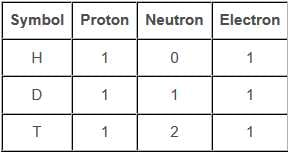2. Write the electronic configuration of any one pair of isotopes and isobars.

12C6 and 14C6 are isotopes, have the same electronic configuration as (2, 4)22Ne10and 22Ne11 are isobars. They have different electronic configuration as given below:
22Ne10 – 2, 8
22Ne11 – 2, 8, 1

Page No: 54

Excercise

1. Compare the properties of electrons, protons and neutrons.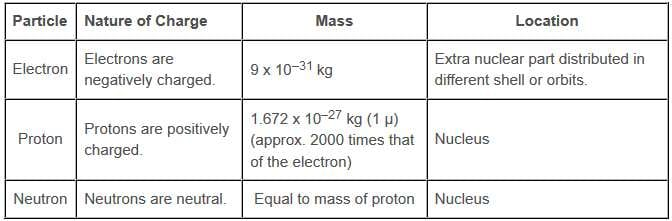2. What are the limitations of J.J. Thomson's model of the atom?

The limitations of J.J. Thomson's model of the atom are:
→ It could not explain the result of scattering experiment performed by rutherford.
→ It did not have any experiment support.

3. What are the limitations of Rutherford's model of the atom?

The limitations of Rutherford's model of the atom are
→ It failed to explain the stability of an atom.
→ It doesn't explain the spectrum of hydrogen and other atoms.

4. Describe Bohr's model of the atom.

→ The atom consists of a small positively charged nucleus at its center.
→ The whole mass of the atom is concentrated at the nucleus and the volume of the nucleus is much smaller than the volume of the atom.
→ All the protons and neutrons of the atom are contained in the nucleus.
→ Only certain orbits known as discrete orbits of electrons are allowed inside the atom.
→ While revolving in these discrete orbits electrons do not radiate energy. These orbits or cells are represented by the letters K, L, M, N etc. or the numbers, n = 1, 2, 3, 4, . . as shown in below figure.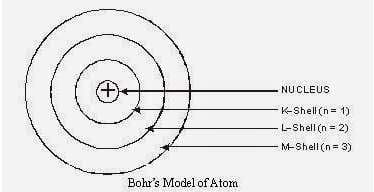5. Compare all the proposed models of an atom given in this chapter.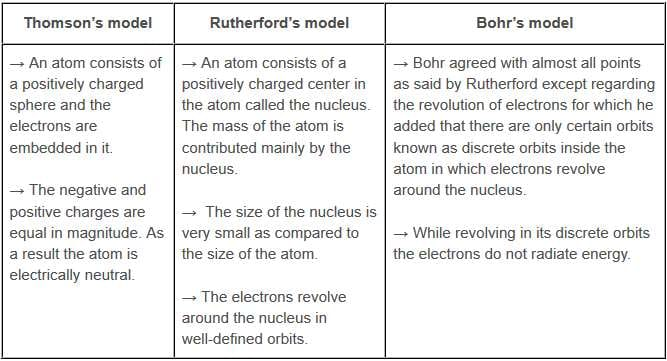6. Summarize the rules for writing of distribution of electrons in various shells for the first eighteen elements.

The rules for writing of the distribution of electrons in various shells for the first eighteen elements are given below.
→ If n gives the number of orbit or energy level, then 2n2 gives the maximum number of electrons possible in a given orbit or energy level. Thus,
First orbit or K-shell will have 2 electrons,
Second orbit or L-shell will have 8 electrons,
Third orbit or M-shell will have 18 electrons.
→ If it is the outermost orbit, then it should have not more than 8 electrons.
→ There should be step-wise filling of electrons in different orbits, i.e., electrons are not accompanied in a given orbit if the earlier orbits or shells are incompletely filled.

7. Define valency by taking examples of silicon and oxygen.

The valency of an element is the combining capacity of that element. The valency of an element is determined by the number of valence electrons present in the atom of that element.→ Valency of Silicon: It has electronic configuration: 2,8,4
Thus, the valency of silicon is 4 as these electrons can be shared with others to complete octet.
→ Valency of Oxygen: It has electronic configuration: 2,6
Thus, the valency of oxygen is 2 as it will gain 2 electrons to complete its octet.

Page No: 55

8. Explain with examples (i) Atomic number, (ii) Mass number, (iii) Isotopes and (iv) Isobars. Give any two uses of isotopes.

(i) Atomic number: The atomic number of an element is the total number of protons present in the atom of that element. For example, nitrogen has 7 protons in its atom. Thus, the atomic number of nitrogen is 7.

(ii) Mass number: The mass number of an element is the sum of the number of protons and neutrons present in the atom of that element. For example, the atom of boron has 5 protons and 6 neutrons. So, the mass number of boron is 5 6 = 11.

(iii) Isotopes: These are atoms of the same element having the same atomic number, but different mass numbers. For example, chlorine has two isotopes with atomic number 17 but mass numbers 35 and 37 represented by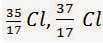(iv) Isobars: These are atoms having the same mass number, but different atomic numbers i.e., isobars are atoms of different elements having the same mass number. For example, Ne has atomic number 10 and sodium has atomic number 11 but both of them have mass numbers as 22 represented by -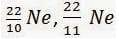Two uses of isotopes:
→ One isotope of uranium is used as a fuel in nuclear reactors.
→ One isotope of cobalt is used in the treatment of cancer.

9. Na  has completely filled K and L shells. Explain.

The atomic number of sodium is 11. So, neutral sodium atom has 11 electrons and its electronic configuration is 2, 8, 1. But Na  has 10 electrons. Out of 10, K-shell contains 2 and L-shell 8 electrons respectively. Thus, Na  has completely filled K and L shells.

10. If bromine atom is available in the form of, say, two isotopes 79 / 35Br (49.7%) and 81 / 35Br (50.3%), calculate the average atomic mass of bromine atom.

It is given that two isotopes of bromine are 79 / 35Br (49.7%) and 81 / 35Br (50.3%). Then, the average atomic mass of bromine atom is given by: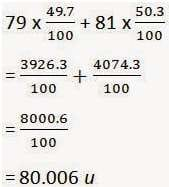11. The average atomic mass of a sample of an element X is 16.2 u. What are the percentages of isotopes 16 / 8 X and 18 / 8 X in the sample?

It is given that the average atomic mass of the sample of element X is 16.2 u.
Let the percentage of isotope 18 / 8 X be y%. Thus, the percentage of isotope 16 / 8 X will be (100 - y) %.

Therefore,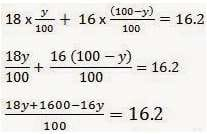18y  1600 - 16y = 1620
2y  1600 = 1620
2y = 1620 - 1600
y= 10
Therefore, the percentage of isotope 18 / 8 X is 10%.
And, the percentage of isotope 16 / 8 X is (100 - 10) % = 90%.

12.  If Z = 3, what would be the valency of the element? Also, name the element.

By Z = 3, we mean that the atomic number of the element is 3. Its electronic configuration is 2, 1. Hence, the valency of the element is 1 (since the outermost shell has only one electron).
Therefore, the element with Z = 3 is lithium.

13.Composition of the nuclei of two atomic species X and Y are given as under

X              Y
Protons =   6              6
Neutrons = 6             8
Give the mass numbers of X and Y. What is the relation between the two species?

Mass number of X = Number of protons Number of neutrons

= 6 6
= 12

Mass number of Y = Number of protons Number of neutrons
= 6 8
= 14

These two atomic species X and Y have the same atomic number, but different mass numbers. Hence, they are isotopes.

14. For the following statements, write T for 'True' and F for 'False'.

(a) J.J. Thomson proposed that the nucleus of an atom contains only nucleons.
? False

(b) A neutron is formed by an electron and a proton combining together. Therefore, it is neutral.
? False

(c) The mass of an electron is about 1 / 2000times that of proton.

? True

(d) An isotope of iodine is used for making tincture iodine, which is used as a medicine.

? False

15. Rutherford's alpha-particle scattering experiment was responsible for the discovery of
(a) Atomic nucleus
(b) Electron
(c) Proton
(d) Neutron
? (a) Atomic nucleus

16. Isotopes of an element have
(a) the same physical properties
(b) different chemical properties
(c) different number of neutrons
(d) different atomic numbers
? (c) different number of neutrons

17. Number of valence electrons in Cl -ion are:
(a) 16
(b) 8
(c) 17
(d) 18
? (b) 8

Page No: 56

18. Which one of the following is a correct electronic configuration of sodium?

(a) 2, 8
(b) 8, 2, 1
(c) 2, 1, 8
(d) 2, 8, 1
? (d) 2, 8, 1

19. Complete the following table.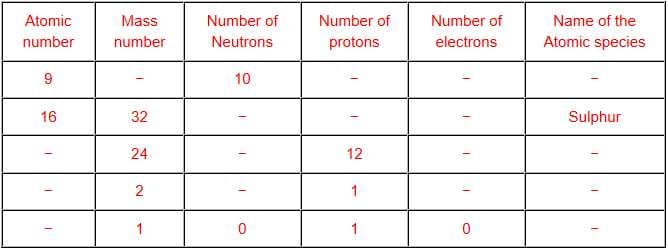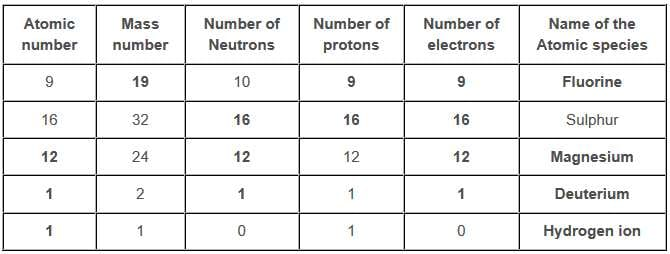The document NCERT Solution - Structure of Atom, Class 9 Science Notes - Class 9 is a part of Class 9 category.
All you need of Class 9 at this link: Class 9Use Code STAYHOME200 and get INR 200 additional OFF

Track your progress, build streaks, highlight & save important lessons and more!

,

,

,

,

,

,

,

,

,

,

,

,

,

,

,

,

,

,

,

,

,

,

,

,

;